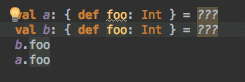{{ message }}

# Missing SymbolOccurence? #1802

Closed
opened this issue Oct 27, 2018 · 1 comment
Closed

# Missing SymbolOccurence?#1802

opened this issue Oct 27, 2018 · 1 comment

###jonas-db commented Oct 27, 2018 • edited

Missing a reference to `doPrint` in `TestClass.a.doPrint()`

``````package yu.examples
class A
trait Tr {
val a = new A {
def doPrint() {
()
}
}
}
object TestClass extends Tr
object Test {
def m(p: => Unit) = 1
def z() = 1
m(TestClass.a.doPrint())
}
``````

## src/main/scala/yu/examples/TestClass.scala

Summary:
Schema => SemanticDB v4
Uri => src/main/scala/yu/examples/TestClass.scala
Text => empty
Language => Scala
Symbols => 10 entries
Occurrences => 20 entries

Symbols:
local1 => method doPrint(): Unit
yu/examples/A# => class A extends AnyRef { +1 decls }
yu/examples/A#`<init>`(). => primary ctor ()
yu/examples/Test. => final object Test extends AnyRef { +2 decls }
yu/examples/Test.m(). => method m(p: => Unit): Int
yu/examples/Test.m().(p) => param p: => Unit
yu/examples/Test.z(). => method z(): Int
yu/examples/TestClass. => final object TestClass extends AnyRef with Tr
yu/examples/Tr# => trait Tr extends AnyRef { +1 decls }
yu/examples/Tr#a. => val method a: A { def doPrint(): Unit }

Occurrences:
[0:8..0:10) => yu/
[0:11..0:19) => yu/examples/
[1:6..1:7) <= yu/examples/A#
[1:7..1:7) <= yu/examples/A#`<init>`().
[2:6..2:8) <= yu/examples/Tr#
[3:8..3:9) <= yu/examples/Tr#a.
[3:16..3:17) => yu/examples/A#
[3:18..3:18) => yu/examples/A#`<init>`().
[4:12..4:19) <= local1
[9:7..9:16) <= yu/examples/TestClass.
[9:25..9:27) => yu/examples/Tr#
[9:27..9:27) => java/lang/Object#`<init>`().
[10:7..10:11) <= yu/examples/Test.
[11:8..11:9) <= yu/examples/Test.m().
[11:10..11:11) <= yu/examples/Test.m().(p)
[11:16..11:20) => scala/Unit#
[12:8..12:9) <= yu/examples/Test.z().
[13:4..13:5) => yu/examples/Test.m().
[13:6..13:15) => yu/examples/TestClass.
[13:16..13:17) => yu/examples/Tr#a.

###olafurpg commented Oct 30, 2018

Thanks for reporting! I am able to reproduce, this is working as expected. Reflective method calls intentionally have no occurrences:

Lines 221 to 222 in c28c65a

 val selectionFromStructuralType = gsym.owner.isRefinementClass if (!selectionFromStructuralType) occurrences(mtree.pos) = symbol

The reason for this behavior is that methods inside structural types cannot have symbols since they are defined by their type. IntelliJ struggles similarly to give sensible meaning for reflective call in "goto definition"